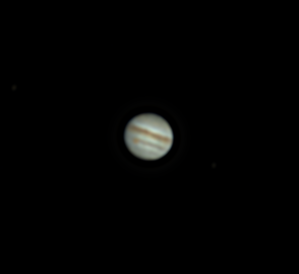Working through the troubleshooting still. Much hardness in troubleshooting as seeing is 1=DISGUSTING.
uninstalled firecapture completely. reinstalled from scratch into new location. Always have the debayer option ON. That seems to have solved the offcolouration of the past few months.
The Great Red Spot is visible in the lower left.
This run was without a barlow lens, so at the native 1800mm focal length

The firecapture 2.6.0.8 log:

Camera=ZWO ASI290MC
Filter=L
Profile=Jupiter
Diameter=37.99″
Magnitude=-2.28
CMI=262.0° CMII=341.7° CMIII=193.3° (during mid of capture)
FocalLength=1650mm
Resolution=0.37″
Filename=2021-12-03-2227_3-L-Jup_ZWO ASI290MC_Exposure=3.0ms.ser
Date=031221
Start=222551.492
Mid=222721.496
End=222851.500
Start(UT)=222551.492
Mid(UT)=222721.496
End(UT)=222851.500
Duration=180.008s
Date_format=ddMMyy
Time_format=HHmmss
LT=UT
Frames captured=12916
File type=SER
Binning=no
ROI=664×608
ROI(Offset)=656×232
FPS (avg.)=71
Shutter=3.045ms
Gain=297 (49%)
WRed=50 (off)
WBlue=90 (off)
Brightness=1 (off)
AutoExposure=off
HighSpeed=off
Gamma=50
SoftwareGain=10 (off)
USBTraffic=68 (off)
AutoHisto=75 (off)
AutoGain=off
FPS=100 (off)
Histogramm(min)=0
Histogramm(max)=193
Histogramm=75%
Noise(avg.deviation)=n/a
Limit=180 Seconds
Sensor temperature=5.8°C